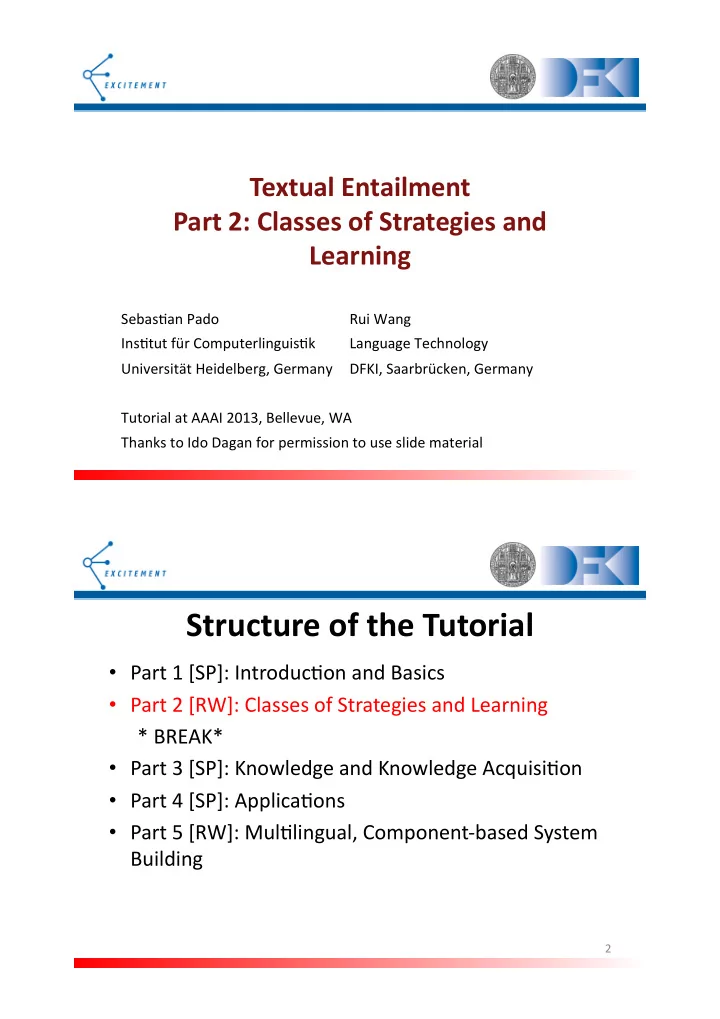# Structure(of(the(Tutorial( Part(1([SP]:(Introduc&on(and(Basics( - PDF document

## Textual(Entailment( Part(2:(Classes(of(Strategies(and( Learning( Sebas&an(Pado ( ( (Rui(Wang( Ins&tut(fr(Computerlinguis&k (Language(Technology( Universitt(Heidelberg,(Germany (DFKI,(Saarbrcken,(Germany(

1. Textual(Entailment( Part(2:(Classes(of(Strategies(and( Learning( Sebas&an(Pado ( ( (Rui(Wang( Ins&tut(für(Computerlinguis&k (Language(Technology( Universität(Heidelberg,(Germany (DFKI,(Saarbrücken,(Germany( Tutorial(at(AAAI(2013,(Bellevue,(WA( Thanks(to(Ido(Dagan(for(permission(to(use(slide(material( Structure(of(the(Tutorial( • Part(1([SP]:(Introduc&on(and(Basics( • Part(2([RW]:(Classes(of(Strategies(and(Learning( (*(BREAK*( • Part(3([SP]:(Knowledge(and(Knowledge(Acquisi&on( • Part(4([SP]:(Applica&ons( • Part(5([RW]:(Mul&lingual,(ComponentYbased(System( Building( 2(

2. MT Triangle ( Meaning Language Language Understanding Generation Direct Source Text Target Text Translation 3( RTE Rectangle ( Meaning Meaning Meaning Inclusion (T) (H) Language Language Understanding Understanding Text Hypothesis Direct Recognition (T) (H) Simplification Simplification Representation Representation Subset (T) (H) 4(

3. Architecture( • Linguis&c(analysis(pipeline((LAP)( • Entailment(decision(algorithm((EDA)( – Classifica&onYbased( – Transforma&onYbased( • Knowledge(base((KB)((next(sec&on)( 5( Architecture( • Linguis&c(analysis(pipeline((LAP)( • Entailment(decision(algorithm((EDA)( – Classifica&onYbased( – Transforma&onYbased( • Knowledge(base((KB)((next(sec&on)( 6(

4. Overview(of(LAPs( • Tokeniza&on((Word(Segmenta&on)( • PartYofYSpeech((POS)(Tagging( • Lemma&za&on( • NamedYEn&ty(Recogni&on( • Syntac&c(Parsing( – Cons&tuent(Parsing( – Dependency(Parsing( • Seman&c(Role(Labeling( • Coreference(Resolu&on( • …( 7( Token@Level(Processing( • Tokeniza&on( – Word(segmenta&on( • Lemma&za&on( Performance( – Morphological(analysis( >97%( • POS(Tagging( • Lexical(Seman&cs( – WordNet,(distribu&onal(similarity,(etc.( 8(

5. An(Example( word(( pos(( lemma(( The(( DT(( the(( TreeTagger(( NP(( TreeTagger(( is(( VBZ(( be(( easy(( JJ(( easy(( to(( TO(( to(( use(( VB(( use(( .(( SENT(( .(( From TreeTagger website 9( ConsBtuents( • Chunking( • NamedYEn&ty(Recogni&on( NER:( 70~90%( • Cons&tuent(Parsing( 10(

6. An(Example( S NP VP . NNP NNP VBD VP VBN NP NP PP NN IN NP NNP NNP [Mr. Todt] PER had been president of [Insilco Corp .] ORG 2: An example from the data of inconsistently labeled named entity and parse struct From Stanford NER (Finkel and Manning, 2009) 11( Dependency( • Syntac&c(Dependency(Parsing( • Seman&c(Dependency(Parsing( Syn:(80~90%( – Seman&c(Role(Labeling( Sem:(75~85%( – PredicateYArgument(Structure( • Logic(Form(Composi&on( 12(

7. An(Example( From MSTParser (McDonald et al., 2005); Visualized by https://code.google.com/p/whatswrong/ 13( An(Example((cont.)( From Laputa SRL (Zhang et al., 2008); Visualized by https://code.google.com/p/whatswrong/ 14(

8. An(Example((cont.)( • H :( Value&is&ques*oned.& is • Syntac&c(dependency( – <is,(SBJ,(value>( VC SBJ A1 – <is,(VC,(ques&oned>( value questioned • Seman&c(dependency( – <ques&oned,(A1,(value>( 15( SemanBc(Roles( • PropBank((Palmer(et(al.,(2005)(and(NomBank((Meyers(et(al.,( 2004)( • Core(arguments:(A0YA5( – different(seman&cs(for(each(verb(( – specified(in(the(PropBank(Frame(files( • 13(types(of(adjuncts(labeled(as(AMY arg (( – where( arg (specifies(the(adjunct(type( 16(

9. Discourse( • Coreference(Resolu&on( • Event(Structure( • Discourse(Parsing( 17( An(Example( explanation-argumentative attribution antithesis reason elaboration-object-attribute-e purpose � � � � � � � [ � Ford Motor Co. and Chrysler Corp. representatives criticized Mr. Tonkin ʼ s plan as unworkable.] [ � It “is going to sound neat to the dealer] [ � except when his 15-day car supply doesn ʼ t include the bright red one] [ � that the lady wants to buy] [ � and she goes up the street] [ � to buy one,”] [ � a Chrysler spokesman said.] From RST Discourse Treebank (Carlson et al., 2002) 18(

10. RTE(Rectangle((more(details)( Meaning Meaning Meaning World Knowledge Inclusion (T) (H) ... Semantic Parsing Language Language Syntactic Parsing Understanding Understanding POS Tagging Tokenization Text Hypothesis Direct Linguistic Knowledge Sentence String Recognition (T) (H) Tokenization Bag-of-Words Simplification Simplification Set-of-Words Content Words ... Representation Representation World Knowledge Subset (T) (H) 19( Overview(of(EDAs( • Classifica&onYbased( – Score(/(Threshold( – Structure(/(Alignment( • Transforma&onYbased( – Edit(distance( – (Knowledge)(rule(applica&on( • MetaYEDA( 20(

11. ClassificaBon((RTE(Style)( Magic Entailment <T, H> Function Non-Entailment 21( Popular(Classifiers( Model( Perceptron/SVM( Naïve(Bayes( LogisBc(Regression( Type( Discrimina&ve( Genera&ve( Discrimina&ve( Distribu&on( N/A( P(X,(Y)( P(Y|X)( Independence( None( Strong( None( Features( Ex/Impilicit( Explicit( Explicit( Speed( Fast/Slow( Fast( Intermediate( 22(

12. Kernel@Based(Methods( • Kernel(Func&on( – Mapping(between(spaces( – CrossYcombina&on(of(features((implicitly!)( – IntroYpair(features(  (crossYpair(features( • Subsequence(Kernel((Lodhi(et(al.,(2002;(Wang(and( Neumann,(2007a)( • Tree(Kernel((Collins(and(Duffy,(2001;(Zanzovo(et(al.,( 2007)( 23( LinguisBc(Features( • Measure(somethingsimilarity(between( t (and( h :(( – Lexical(overlap((unigram,(NYgram,(subsequence)( • Assisted(by(lexical(resources(like(WordNet( – Syntac&c(matching( – LexicalYsyntac&c(varia&ons((“paraphrases”)( – Seman&c(role(matching( – Global(similarity(parameters((e.g.(nega&on,(modality)( • Detect(mismatch((for(nonYentailment)( 24(

13. Data(Structures( • StringYtoYString(rewri&ng( – String(edit(distance((MacCartney(and(Manning,(2007)( – Tree(skeleton(difference((Wang(and(Neumann,(2007a)( • TreeYtoYTree(edi&ng( – Tree(edit(distance((Kouylekov(and(Magnini,(2005)( • GraphYtoYGraph(mapping( – Graph(matching((Haghighi(et(al.,(2005)( 25( Word(Overlap( • |T|:(number(of(words(in(T( • |H|:(number(of(words(in(H( • E 1 (=(|T � H|(/(|H|( 57.2&on& • E 2 (=(|T � H|(/(|T|( average& • E 3 (=((2(*(E 1 (*(E 2 )(/(E 1 (+(E 2( • Content(words(only( • Lemma&za&on( From (Mehdad and Magnini, 2009) 26(

14. Dependencies( • Syntac&c(dependency(trees( – Dependency(triples( <Node,&Rela*on,&Head>& – Bag(of(such(triples( • E 1 � (=(|Triple(T) � Triple((H)|(/(|Triple(H)|( 27( Dependencies((cont.)( From (Wang and Zhang, 2009) 28(

15. Results((RTE@5)( • DFKI1:(BoW(and(syntac&c(dependency( • DFKI2:(BoW,(syntac&c,(and(seman&c(dependency( • DFKI3:(BoW(and(joint(syntac&c(and(seman&c( representa&on( From (Wang et al., 2009) 29( Larger(Sub@Structures( • Dependency(paths( – Common(subYpaths( • Subtrees( • E 1 �� =(|Subtree(T) � Subtree(H)|(/(|Subtree(H)|( 30(

16. Subtrees( T 1 ⇒ H 1 T 2 ⇒ H 2 T 1 “Farmers feed cows animal extracts” T 2 “They feed dolphins fish” H 1 “Cows eat animal extracts” H 2 “Fish eat dolphins” feed → eat X Y X Y T 3 ⇒ H 3 T 3 “Mothers feed babies milk” H 3 “Babies eat milk” From (Zanzotto and Dell'Arciprete, 2009) 31( Tree(Skeletons( • T:( Doctor&Robin&Warren&and& • H:( Robin&Warren&was& Barry&Marshall&received&Nobel& awarded&a&Nobel&Prize.& Prize&…& From (Wang and Neumann, 2007) 32(

17. Results( • RTEY2( BagYofYWords( Triple( Similarity( Similarity( Tree(Skeleton( Matcher( • RTEY3( From (Wang, 2007) 33( The(RIGHT(level( Meaning �������� �������������� � � � ����� � Text ������������������� � • TradeYoffs(between( – Competence (of(the(knowledge((deeper)( – Performance (of(the(processing((shallower)( 34(

18. Alignment@Based(Approaches( • Word(alignment((Glickman(et(al.,(2006)( • Phrase(alignment((chambers(et(al.,(2007;( MacCartney(et(al.,(2008)( • Rela&on(alignment((Sammons(et(al.,(2009)( 35( Overview(of(EDAs( • Classifica&onYbased( – Score(/(Threshold( – Structure(/(Alignment( • Transforma&onYbased( – Edit(distance( – (Knowledge)(rule(applica&on( • MetaYEDA( 36(

19. Matching(vs.(TransformaBons( • Direct(matching((so(far,(no(chaining)( • Sequence(of(transforma&ons((A(proof)( T(=(T 0 (→(T 1 (→(T 2 (→(...(→(T n (=(H( – TreeYEdits( – Knowledge(based(Entailment(Rules( 37( Edit(Distance( • (Limited)(preYdefined(operators( – Inser&on( Weakly& – Dele&on( linguis*cally& – Subs&&on( mo*vated!& • StringYtoYString( • TreeYtoYTree( • The(EDITS(system((Kouylekov(and(Negri,(2010)( – Es&mate(confidence(in(each(opera&on( • Wang(and(Manning((2010),(Heilman(and(Smith((2010),(etc.( 38(

More recommend